Derive the Quadratic Formula MathBitsNotebook.com Terms of Use   Contact Person: Donna Roberts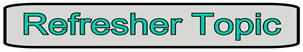The process of completing the square will show us how the quadratic formula was developed from solving a quadratic equation.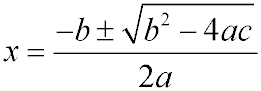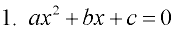1. Start with a quadratic equation set = 0.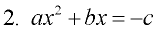2. Move the constant to the right side.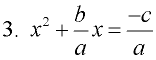3. Divide each term by a to get the leading coefficient to be one.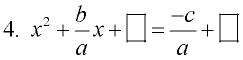4. Get ready for a perfect square trinomial on the left side (put in the boxes).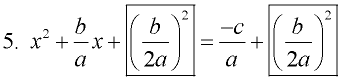5. To find the needed value, take half of the coefficient of the x-term,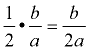, and square it,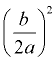. Add this value to both sides of the equation (put it in the boxes).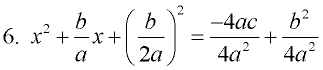6. Square the last term on the right side. Find a common denominator on the right.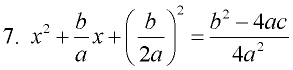7. Combine terms on the right, placing b2 in front of -4ac in the numerator.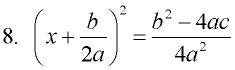8. Re-write the perfect square trinomial on the left side as the square of a binomial.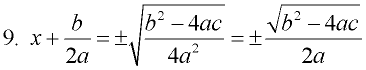9. Take the square root of both sides. Simplify the radical on the right side.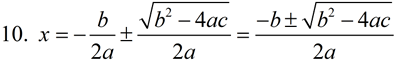10. Subtract the constant away from x. Write as a single fraction on the right side.By completing the square, we discovered the quadratic formula!!